##### Common Core Math For Parents For Dummies with Videos Online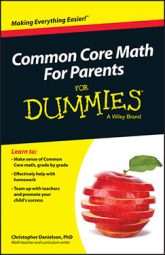In Common Core fifth grade math, students build on what they learned earlier about whole‐number place value, as they begin to study decimal place value — that is, they study digits to the right of the decimal point.

One of the most challenging things about studying decimal place value is that several of the ways of thinking about whole numbers that children commonly adopt don't apply to numbers to the right of the decimal point. Here are two examples where decimals behave differently from the expectations many students have based on their experiences with whole numbers. (These examples illustrate why it's important not to focus on rules, and why it's important instead to focus on meaning.)

• For whole numbers, the number with more digits is larger. With decimals, the number with more digits may be smaller. When you compare two whole numbers — say, 235 and 62 — the one with more digits is larger. That's because the 2 in 235 is in the hundreds place, while 62 has no hundreds at all. But with decimals, the story is different. 0.235 isn't larger than 0.62. Every time you add a digit to the right of a number, you add a smaller place value — this is the opposite of the process when you add digits to the left of the decimal point.

Students need to work in ways that keep their focus on the meaning of the places they work with, not just on generating new rules for this new environment. If students form bad habits with decimals, these habits prove remarkably robust and difficult to overcome. You will likely see your fifth grader decomposing decimals (for example, writing 0.62 as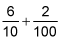and drawing pictures in order to focus on the meanings of the numbers they work with rather than on memorizing a new set of rules.

• In order to multiply by 10, you can add a zero on the end of the whole number. When you multiply decimals by 10, the digits move one place to the left. When you multiply 42 by 10, you get 420 — the same digits, but with a 0 on the end. This fact makes multiplication by 10 much easier than multiplication by (for example) 9. It's an important rule that makes multiplication algorithms possible. But it isn't true for numbers to the right of the decimal point.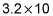isn't equal to 3.20.

Multiplying decimals by 10 moves the digits one place to the left because now they represent an amount 10 times larger than before. In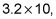the 3 moves from the ones place to the tens place and the 2 moves from the tenths place to the ones place to be 32. This is actually the same thing that goes on when you multiply whole numbers too. When you multiply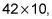the 4 moves from the tens place to the hundreds place and the 2 moves from the ones place to the tens place to be 420. But then the ones place is empty, so you fill that place with a 0. As always in the Common Core Standards, the focus is on the meaning of the numbers, not on learning and applying rules without meaning.